# Ideal Gas Law Gas Stoichiometry Ideal Gas Law

• Slides: 11Ideal Gas Law & Gas Stoichiometry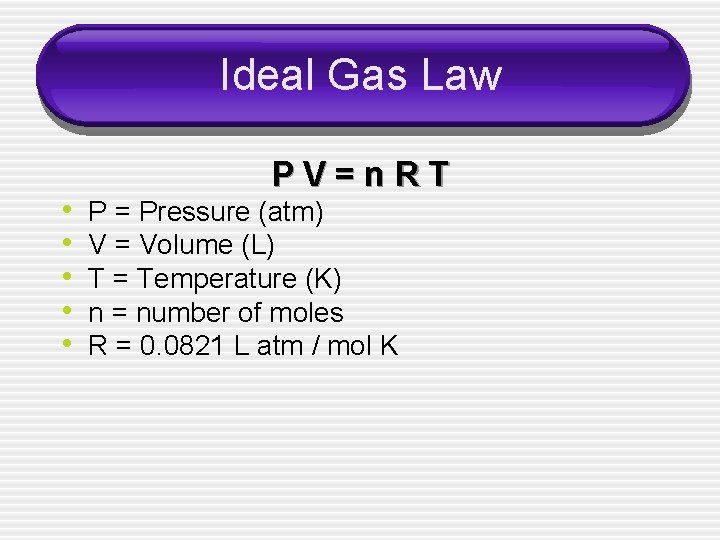Ideal Gas Law • • • PV=n. RT P = Pressure (atm) V = Volume (L) T = Temperature (K) n = number of moles R = 0. 0821 L atm / mol K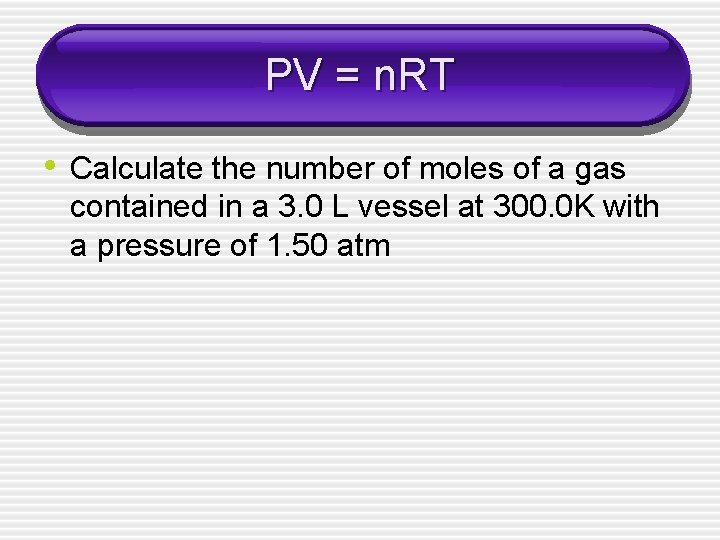PV = n. RT • Calculate the number of moles of a gas contained in a 3. 0 L vessel at 300. 0 K with a pressure of 1. 50 atm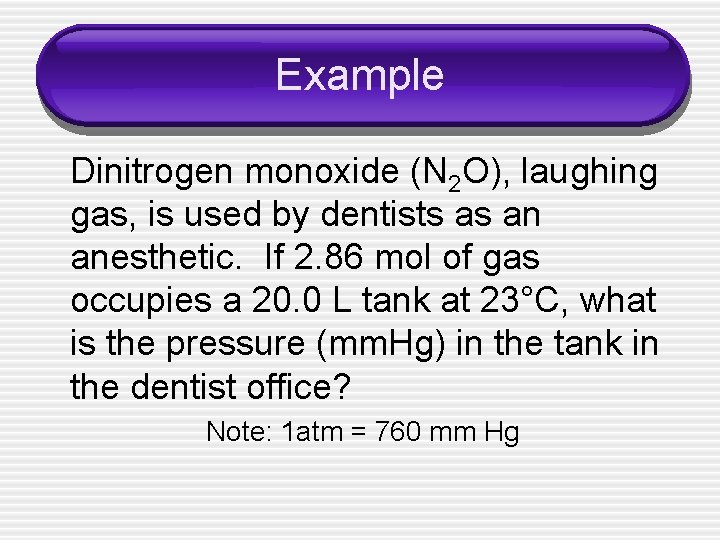Example Dinitrogen monoxide (N 2 O), laughing gas, is used by dentists as an anesthetic. If 2. 86 mol of gas occupies a 20. 0 L tank at 23°C, what is the pressure (mm. Hg) in the tank in the dentist office? Note: 1 atm = 760 mm HgPermutations of the Ideal Gas Law • • • PV = m. RT M P = Pressure (atm) V = volume (L) m = mass of the gas (g) R = 0. 0821 L atm / mol K T = Temperature (K) M = molecular mass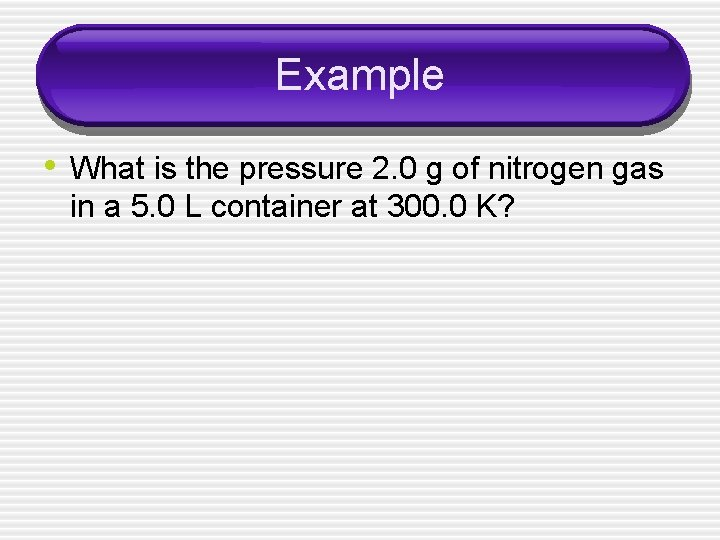Example • What is the pressure 2. 0 g of nitrogen gas in a 5. 0 L container at 300. 0 K?Permutations of the Ideal Gas Law • • • P = DRT M P = pressure (atm) D = density (g/L) R = 0. 0821 L atm / mol K T = temperature (K) M = molecular massExample • What is the molar mass of a gas that has a density of 1. 40 g/L at STP? § NOTE – STP is standard temperature and pressure § At STP temperature is 273 K and pressure is 1. 00 atm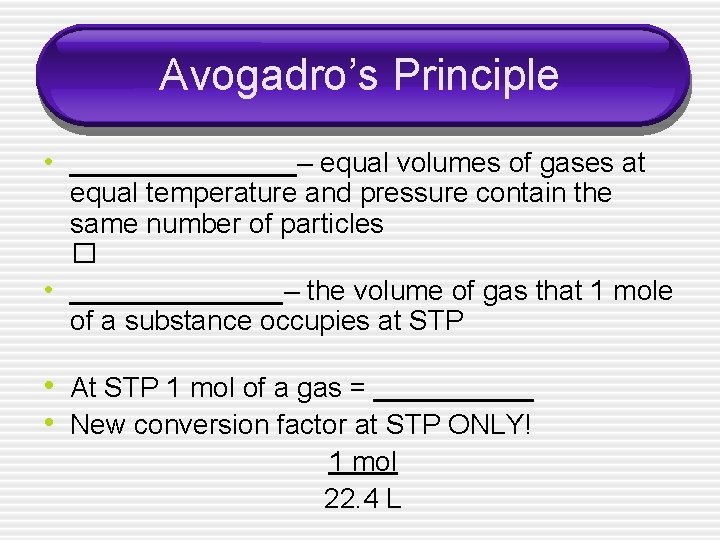Avogadro’s Principle • _________– equal volumes of gases at equal temperature and pressure contain the same number of particles � • ________– the volume of gas that 1 mole of a substance occupies at STP • At STP 1 mol of a gas = ______ • New conversion factor at STP ONLY! 1 mol 22. 4 LExample • Calculate the volume 0. 881 mol of a gas will occupy at STP.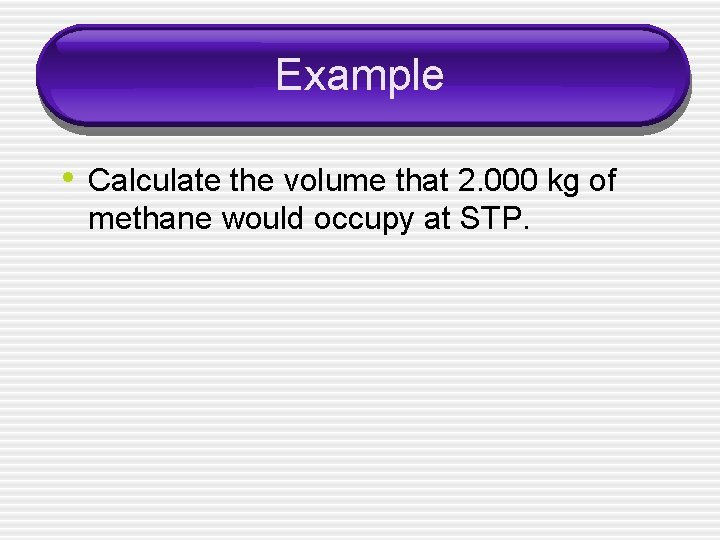Example • Calculate the volume that 2. 000 kg of methane would occupy at STP.